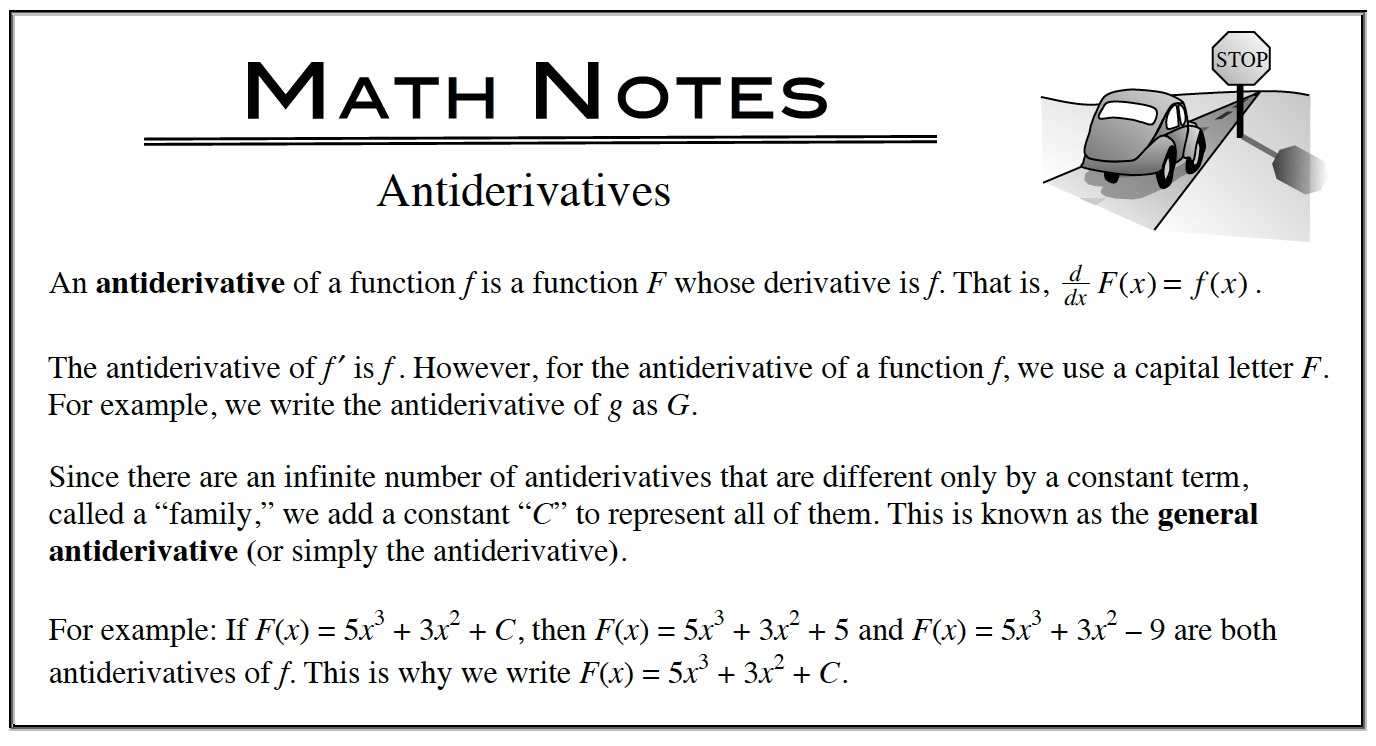Home > APCALC > Chapter 7 > Lesson 7.2.4 > Problem7-91

7-91.

Write a possible equation for y given $\frac { d y } { d x }= 12e^{4x} −\frac{1}{x}$ . Homework Help ✎

You know the derivative but you want to know the original function. What should you do?